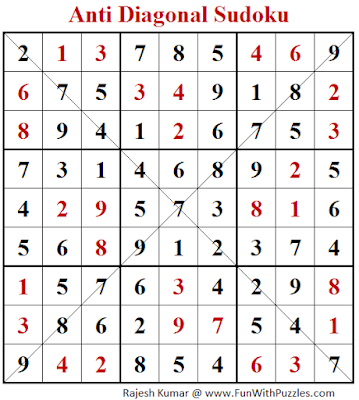Double Diagonal Sudoku is a very interesting Diagonal Sudoku variation. In Diagonal Sudoku we have two main diagonals of size 9 on which no number can repeat. It means that all numbers from 1 to 9 are used exactly once on these diagonal. However, in Double Diagonal Sudoku, there are four diagonals of size 8 on which numbers cannot repeat. It means that each of the four diagonals contains only 8 numbers and one number goes missing in each diagonal. It is very interesting to find that which number is missing in these diagonals and sometimes finding the missing number from the diagonal itself helps to solve this puzzle very fast. This is my first Double Diagonal Sudoku on this blog and I am looking forward to creating more Sudoku puzzles of this Sudoku type soon.
This Double Diagonal Sudoku, I am posting in Fun With Sudoku Series as 184th puzzle in this series. Can you solve this Double Diagonal Sudoku puzzle?
Rules of Double Diagonal Sudoku
Apply Standard Classic Sudoku rules. Additionally, the digits 1 to 9 cannot repeat in any of the four eight-cell diagonals drawn on the grid.
Next Sudoku Serbian Frame Sudoku (Fun With Sudoku #185)

Below is the solution of Anti Diagonal Sudoku Puzzle, which is published with the title Anti Diagonal Sudoku Puzzle (Fun With Sudoku #247)Anti Diagonal Sudoku (Fun With Sudoku #247) Puzzle Solution

#### 1 comment:

Anonymous said...

Incorrect solution posted for anti-diagonal #247 - in the bottom middle square there is a four where there should be a 1. Fun puzzle!